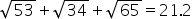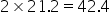Mathematics
Easy

Question

# If the midpoints of each side of a triangle are (5, 2), (12, 4), (9, 9) and forms a triangle. Find the perimeter of the original triangle

## 40.541.342.443.5Hint:

## The correct answer is: 42.4

### Perimeter = AB + BC +AC =Perimeter of original triangle =Linear equation in one variable can be solved using fundamental operations

### Related Questions to study#### With Turito Foundation.#### Get an Expert Advice From Turito.